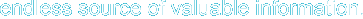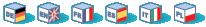# 6. Output functions

I guess you can't wait any more to see some action. Back to our odyssey of curses functions. Now that curses is initialized, let's interact with world.

There are three classes of functions which you can use to do output on screen.

1. addch() class: Print single character with attributes

2. printw() class: Print formatted output similar to printf()

These functions can be used interchangeably and it's a matter of style as to which class is used. Let's see each one in detail.

## 6.1. addch() class of functions

These functions put a single character into the current cursor location and advance the position of the cursor. You can give the character to be printed but they usually are used to print a character with some attributes. Attributes are explained in detail in later sections of the document. If a character is associated with an attribute(bold, reverse video etc.), when curses prints the character, it is printed in that attribute.

In order to combine a character with some attributes, you have two options:

• By OR'ing a single character with the desired attribute macros. These attribute macros could be found in the header file ncurses.h. For example, you want to print a character ch(of type char) bold and underlined, you would call addch() as below.  ` addch(ch | A_BOLD | A_UNDERLINE);`

• By using functions like attrset(),attron(),attroff(). These functions are explained in the Attributes section. Briefly, they manipulate the current attributes of the given window. Once set, the character printed in the window are associated with the attributes until it is turned off.

Additionally, curses provides some special characters for character-based graphics. You can draw tables, horizontal or vertical lines, etc. You can find all avaliable characters in the header file ncurses.h. Try looking for macros beginning with ACS_ in this file.

mvaddch() is used to move the cursor to a given point, and then print. Thus, the calls:
 ``` move(row,col); /* moves the cursor to rowth row and colth column */ addch(ch);```
can be replaced by
 ` mvaddch(row,col,ch);`

In a similar fashion mvwaddch() function is used to add a character into the given window at the given coordinates.

Now, we are familiar with the basic output function addch(). But, if we want to print a string, it would be very annoying to print it character by character. Fortunately, ncurses provides printf-like or puts-like functions.

## 6.3. printw() class of functions

These functions are similar to printf() with the added capability of printing at any position on the screen.

### 6.3.1. printw() and mvprintw

These two functions work much like printf(). mvprintw() can be used to move the cursor to a position and then print. If you want to move the cursor first and then print using printw() function, use move() first and then use printw() though I see no point why one should avoid using mvprintw(), you have the flexibility to manipulate.

### 6.3.2. wprintw() and mvwprintw

These two functions are similar to above two except that they print in the corresponding window given as argument.

### 6.3.3. vwprintw()

This function is similar to vprintf(). This can be used when variable number of arguments are to be printed.

### 6.3.4. A Simple printw example

Example 3. A Simple printw example

 ```#include /* ncurses.h includes stdio.h */ #include int main() { char mesg[]="Just a string"; /* message to be appeared on the screen */ int row,col; /* to store the number of rows and * * the number of colums of the screen */ initscr(); /* start the curses mode */ getmaxyx(stdscr,row,col); /* get the number of rows and columns */ mvprintw(row/2,(col-strlen(mesg))/2,"%s",mesg); /* print the message at the center of the screen */ mvprintw(row-2,0,"This screen has %d rows and %d columns\n",row,col); printw("Try resizing your window(if possible) and then run this program again"); refresh(); getch(); endwin(); return 0; }```

Above program demonstrates how easy it is to use printw. You just feed the coordinates and the message to be appeared on the screen, then it does what you want.

The above program introduces us to a new function getmaxyx(), a macro defined in ncurses.h. It gives the number of columns and the number of rows in a given window. getmaxyx() does this by updating the variables given to it. Since getmaxyx() is not a function we don't pass pointers to it, we just give two integer variables.

## 6.4. addstr() class of functions

addstr() is used to put a character string into a given window. This function is similar to calling addch() once for each character in a given string. This is true for all output functions. There are other functions from this family such as mvaddstr(),mvwaddstr() and waddstr(), which obey the naming convention of curses.(e.g. mvaddstr() is similar to the respective calls move() and then addstr().) Another function of this family is addnstr(), which takes an integer parameter(say n) additionally. This function puts at most n characters into the screen. If n is negative, then the entire string will be added.

## 6.5. A word of caution

All these functions take y co-ordinate first and then x in their arguments. A common mistake by beginners is to pass x,y in that order. If you are doing too many manipulations of (y,x) co-ordinates, think of dividing the screen into windows and manipulate each one separately. Windows are explained in the windows section.

Hosting by: Hurra Communications Ltd.
Generated: 2007-01-26 17:57:45